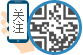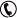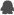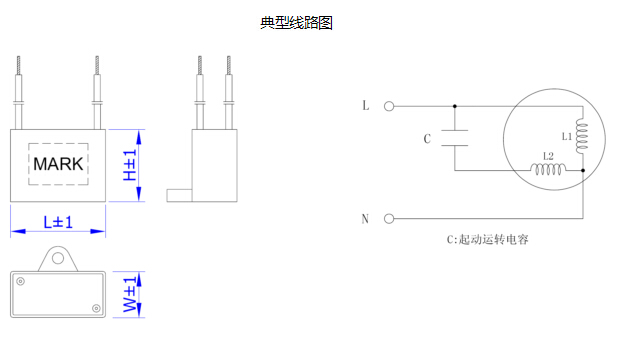﻿ CBB61 交流电容器 双线系列 艾美特 美的 格力 指定供应商

# 中国家电业薄膜电容品牌电话：400-9903-638QQ：304178812

## CBB61 交流电容器 双线系列

• 产品简介：采用电工级聚丙烯薄膜作为介质、锌铝金属化层作为电极,优质工程塑料外壳，阻燃环氧树脂灌封， PVC电子线引出，通用性强，装配灵活。

• ### 联系我们

CBB61 交流电容器 双线系列  艾美特 美的 格力 指定供应商

采用电工级聚丙烯薄膜作为介质、锌铝金属化层作为电极,优质工程塑料外壳，阻燃环氧树脂灌封，PVC电子线引出，

通用性强，装配灵活。认证标志     Safety approvals UL﹑VDE﹑CQC﹑TUV 执行标准     Implemented standard GB/T3667.1、UL810、EN60252-1 环境温度     Environment temperature -40℃~+105℃ 额定电压     Rated voltage 110VAC~600VAC (50/60Hz) 容量范围     Capacitance range 0.1μF～80μF 容量偏差     Capacitance tolerance ±5%（J）、±10%（K） 损耗角正切     Tangent of loss angle(20℃) ≤0.002  (100Hz ) 试验电压      Testing voltage 极间 between terminals 2*UN (VAC)/60s(CQC、VDE、TUV) ,  1.5*UN (VAC)/60s (UL) 极壳 between terminals and case 2*UN+1000（VAC）/ 60s(≥2000VAC) 防护等级    Class of safety protection CBB61 :P0 class ; CBB61-P2: P2 class 故障电流      Fault current CBB61-P2 : 10,000AFC ( UL 810)

常用规格(Dimension)                                   unitmm

 Rated  Cap. 250VAC 300VAC 350VAC 400VAC 450VAC 500VAC 550～600VAC L×W×H L×W×H L×W×H L×W×H L×W×H L×W×H L×W×H 0.40μF —— —— —— —— 31×9×17 31×9×19 32×11×21 0.50μF —— —— —— —— 31×9×17 31×9×19 32×11×21 0.56μF —— —— —— 31×9×17 31×9×19 32×10×19.5 32×11×21 0.60μF —— —— —— 31×9×17 32×10×19.5 32×11×21 36×11×22 0.80μF —— —— 31×9×19 32×10×19.5 32×11×21 36×10.5×20 36×12×22 1.0μF —— 31×9×7 32×10×19.5 32×11×21 36×10.5×20 36×11×22 36×12×22 1.2μF 31×9×17 32×10×19.5 32×11×21 36×11×22 36×12×22 36×12.5×24 36×15×25 1.5μF 31×9×19 32×11×21 36×11×22 36×12×22 36×12.5×24 36×15×25 36×16×28 1.8μF 31×10×19 36×10.5×20 36×12×22 36×12.5×24 36×15×25 36×16×28 38×18.5×29 2.0μF 31×10×19 36×11×22 36×12.5×24 37×13.5×24 36×15×25 38×17×28 38×19.5×31 2.2μF 32×11×21 36×11×22 36×12.5×24 37×13.5×24 36×16×28 38×18.5×29 38×19.5×31 2.5μF 32×11×21 36×12×22 36×15×25 36×16×28 38×17×28 38×19.5×31 48×19×28 3.0μF 36×12×22 36×12.5×24 36×16×28 38×17×28 38×19.5×31 47×17×31 47×20×31 3.5μF 36×12×22 36×15×25 38×17×28 38×18.5×29 47×17×28 47×20×31 47×23×32 4.0μF 36×12.5×24 38×14.5×27 38×18.5×29 38×19.5×31 48×19×28 47×20×33 47×23×35 4.5μF 36×12.5×24 36×16×28 38×19.5×31 48×17×28 47×20×31 47×23×35 47×24×37 5.0μF 37×13.5×24 38×17×28 48×17×28 47×17×31 47×20×31 47×23×35 47×27×37 6.0μF 38×16×27 38×18.5×29 38×19.5×31 47×20×31 47×23×35 47×27×37 58×26×38 6.5μF 38×16×27 38×18.5×29 47×19×31 48×22×32 47×23×35 48×28×40 58×26×38 8.0μF 48×17×28 48×19×28 47×23×32 47×23×35 47×27×37 58×23×38 58×30×40 10μF 47×20×31 47×20×33 47×24×37 48×28×40 58×26×38 58V30×40 70×38×52 12μF 48×19×28 47×23×35 48×28×40 58×26×38 58×28×40 58V32×43 70×38×52

•